Topics: AI | Data Science | Data Visualization | Deep Learning | Machine Learning | NLP | Python | R | Statistics

KDnuggets Home » News » 2017 » Nov » Tutorials, Overviews » The 10 Statistical Techniques Data Scientists Need to Master ( 17:n45 )

#The 10 Statistical Techniques Data Scientists Need to Master

The author presents 10 statistical techniques which a data scientist needs to master. Build up your toolbox of data science tools by having a look at this great overview post.

6 — Dimension Reduction:

Dimension reduction reduces the problem of estimating p + 1 coefficients to the simple problem of M + 1 coefficients, where M < p. This is attained by computing M different linear combinations, or projections, of the variables. Then these M projections are used as predictors to fit a linear regression model by least squares. 2 approaches for this task are principal component regression and partial least squares.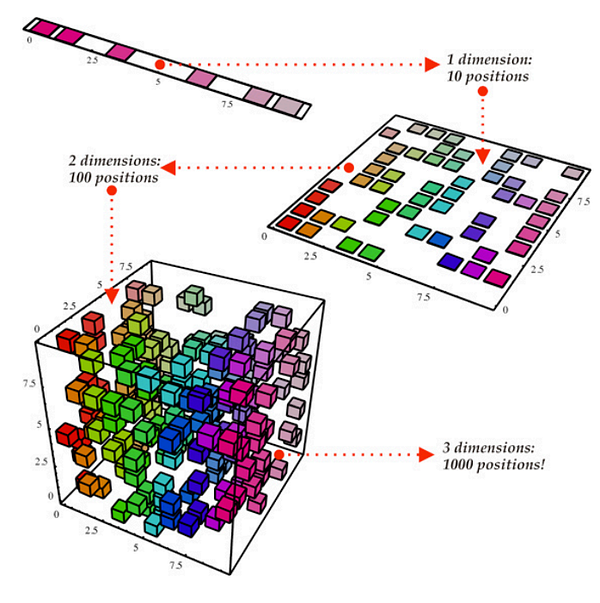• One can describe Principal Components Regression as an approach for deriving a low-dimensional set of features from a large set of variables. The firstprincipal component direction of the data is along which the observations vary the most. In other words, the first PC is a line that fits as close as possible to the data. One can fit p distinct principal components. The second PC is a linear combination of the variables that is uncorrelated with the first PC, and has the largest variance subject to this constraint. The idea is that the principal components capture the most variance in the data using linear combinations of the data in subsequently orthogonal directions. In this way, we can also combine the effects of correlated variables to get more information out of the available data, whereas in regular least squares we would have to discard one of the correlated variables.
• The PCR method that we described above involves identifying linear combinations of X that best represent the predictors. These combinations (directions) are identified in an unsupervised way, since the response Y is not used to help determine the principal component directions. That is, the response Y does not supervise the identification of the principal components, thus there is no guarantee that the directions that best explain the predictors also are the best for predicting the response (even though that is often assumed). Partial least squares (PLS) are a supervised alternative to PCR. Like PCR, PLS is a dimension reduction method, which first identifies a new smaller set of features that are linear combinations of the original features, then fits a linear model via least squares to the new M features. Yet, unlike PCR, PLS makes use of the response variable in order to identify the new features.

7 — Nonlinear Models:

In statistics, nonlinear regression is a form of regression analysis in which observational data are modeled by a function which is a nonlinear combination of the model parameters and depends on one or more independent variables. The data are fitted by a method of successive approximations. Below are a couple of important techniques to deal with nonlinear models:

• A function on the real numbers is called a step function if it can be written as a finite linear combination of indicator functions of intervals. Informally speaking, a step function is a piecewise constant function having only finitely many pieces.
• piecewise function is a function which is defined by multiple sub-functions, each sub-function applying to a certain interval of the main function’s domain. Piecewise is actually a way of expressing the function, rather than a characteristic of the function itself, but with additional qualification, it can describe the nature of the function. For example, a piecewise polynomial function is a function that is a polynomial on each of its sub-domains, but possibly a different one on each.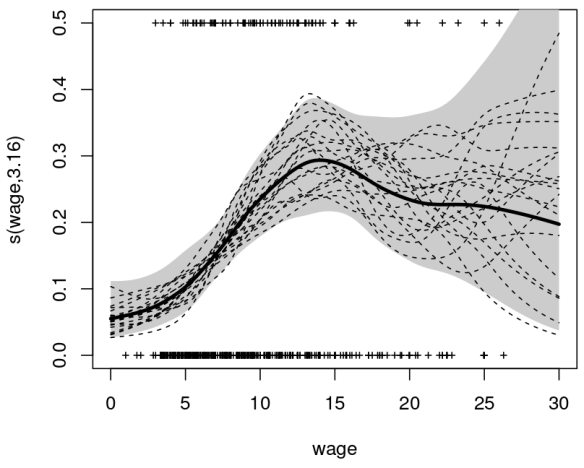• spline is a special function defined piecewise by polynomials. In computer graphics, spline refers to a piecewise polynomial parametric curve. Splines are popular curves because of the simplicity of their construction, their ease and accuracy of evaluation, and their capacity to approximate complex shapes through curve fitting and interactive curve design.
• generalized additive modelis a generalized linear model in which the linear predictor depends linearly on unknown smooth functions of some predictor variables, and interest focuses on inference about these smooth functions.

8 — Tree-Based Methods:

Tree-based methods can be used for both regression and classification problems. These involve stratifying or segmenting the predictor space into a number of simple regions. Since the set of splitting rules used to segment the predictor space can be summarized in a tree, these types of approaches are known as decision-tree methods. The methods below grow multiple trees which are then combined to yield a single consensus prediction.

• Bagging is the way decrease the variance of your prediction by generating additional data for training from your original dataset using combinations with repetitions to produce multistep of the same carnality/size as your original data. By increasing the size of your training set you can’t improve the model predictive force, but just decrease the variance, narrowly tuning the prediction to expected outcome.
• Boosting is an approach to calculate the output using several different models and then average the result using a weighted average approach. By combining the advantages and pitfalls of these approaches by varying your weighting formula you can come up with a good predictive force for a wider range of input data, using different narrowly tuned models.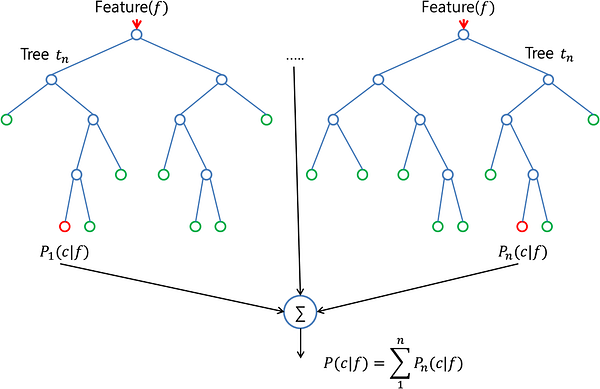• The random forest algorithm is actually very similar to bagging. Also here, you draw random bootstrap samples of your training set. However, in addition to the bootstrap samples, you also draw a random subset of features for training the individual trees; in bagging, you give each tree the full set of features. Due to the random feature selection, you make the trees more independent of each other compared to regular bagging, which often results in better predictive performance (due to better variance-bias trade-offs) and it’s also faster, because each tree learns only from a subset of features.

9 — Support Vector Machines: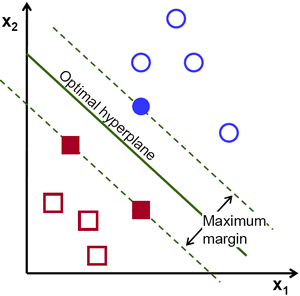SVM is a classification technique that is listed under supervised learning models in Machine Learning. In layman’s terms, it involves finding the hyperplane (line in 2D, plane in 3D and hyperplane in higher dimensions. More formally, a hyperplane is n-1 dimensional subspace of an n-dimensional space) that best separates two classes of points with the maximum margin. Essentially, it is a constrained optimization problem where the margin is maximized subject to the constraint that it perfectly classifies the data (hard margin).

The data points that kind of “support” this hyperplane on either sides are called the “support vectors”. In the above picture, the filled blue circle and the two filled squares are the support vectors. For cases where the two classes of data are not linearly separable, the points are projected to an exploded (higher dimensional) space where linear separation may be possible. A problem involving multiple classes can be broken down into multiple one-versus-one or one-versus-rest binary classification problems.

10 — Unsupervised Learning:

So far, we only have discussed supervised learning techniques, in which the groups are known and the experience provided to the algorithm is the relationship between actual entities and the group they belong to. Another set of techniques can be used when the groups (categories) of data are not known. They are called unsupervised as it is left on the learning algorithm to figure out patterns in the data provided. Clustering is an example of unsupervised learning in which different data sets are clustered into groups of closely related items. Below is the list of most widely used unsupervised learning algorithms: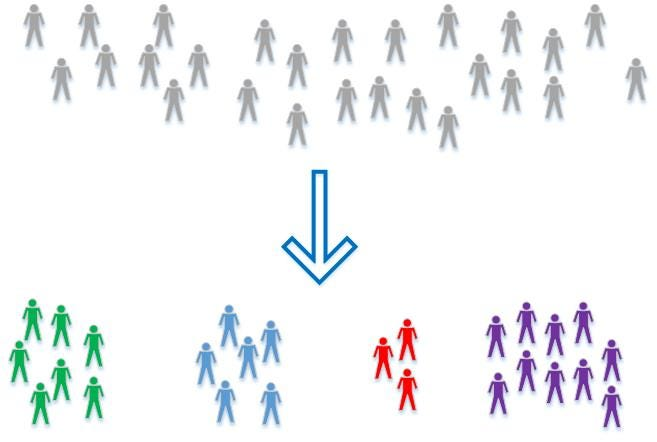• Principal Component Analysis helps in producing low dimensional representation of the dataset by identifying a set of linear combination of features which have maximum variance and are mutually un-correlated. This linear dimensionality technique could be helpful in understanding latent interaction between the variable in an unsupervised setting.
• k-Means clustering: partitions data into k distinct clusters based on distance to the centroid of a cluster.
• Hierarchical clustering: builds a multilevel hierarchy of clusters by creating a cluster tree.

This was a basic run-down of some basic statistical techniques that can help a data science program manager and or executive have a better understanding of what is running underneath the hood of their data science teams. Truthfully, some data science teams purely run algorithms through python and R libraries. Most of them don’t even have to think about the math that is underlying. However, being able to understand the basics of statistical analysis gives your teams a better approach. Have insight into the smallest parts allows for easier manipulation and abstraction. I hope this basic data science statistical guide gives you a decent understanding!

P.S: You can get all the lecture slides and RStudio sessions from my GitHub source code here. Thanks for the overwhelming response!

Bio: James Le is currently applying for Master of Science Computer Science programs in the US for the Fall 2018 admission. His intended research will focus on Machine Learning and Data Mining. In the mean time, he is working as a freelance full-stack web developer.

Original. Reposted with permission.

Related:

Top Stories Past 30 Days
Most SharedGet KDnuggets, a leading newsletter on AI, Data Science, and Machine Learning